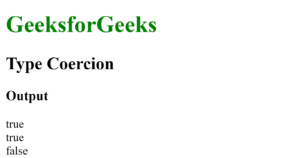Related Articles
What is Type Coercion in JavaScript ?
• Last Updated : 01 Jul, 2020

Type Coercion refers to the process of automatic or implicit conversion of values from one data type to another. This includes conversion from Number to String, String to Number, Boolean to Number etc. when different types of operators are applied to the values.

In case the behavior of the implicit conversion is not sure, the constructors of a data type can be used to convert any value to that datatype, like the Number(), String() or Boolean() constructor.

1. String to Number Conversion: When any string or non-string value is added to a string, it always converts the non-string value to a string implicitly. When the string ‘Rahul’ is added to the number 10 then JavaScript does not give an error. It converts the number 10 to string ’10’ using coercion and then concatenates both the strings. Some more examples are shown below.

Example:

 ` ` ` ` ` `  ` ` `    `` ` `        ``What is Type Coercion  ` `        ``in` `JavaScript? ` `    `` ` ` ` ` `  ` ` `    ``

` `        ``GeeksforGeeks ` `    ``

` `     `  `    ``

Type Coercion

` `     `  `    ``

Output

` `     `  `    `` ` ` ` ` `  ` `

Output:2. String to Number Conversion: When an operation like subtraction (-), multiplication (*), division (/) or modulus (%) is performed, all the values that are not number are converted into the number data type, as these operations can be performed between numbers only. Some examples of this are shown below.

Example:

 ` ` ` ` ` `  ` ` `    `` ` `        ``What is Type Coercion ` `        ``in` `JavaScript? ` `    `` ` ` ` ` `  ` ` `    ``

` `        ``GeeksforGeeks ` `    ``

` ` `  `    ``

Type Coercion

` ` `  `    ``

Output

` ` `  `    `` ` ` ` ` `  ` `

Output:3. Boolean to Number: When a Boolean is added to a Number, the Boolean value is converted to a number as it is safer and easier to convert Boolean values to Number values. A Boolean value can be represented as 0 for ‘false’ or 1 for ‘true’. Some examples of this are shown below.

Example:

 ` ` ` ` ` `  ` ` `    `` ` `        ``What is Type Coercion ` `        ``in` `JavaScript? ` `    `` ` ` ` ` `  ` ` `    ``

` `        ``GeeksforGeeks ` `    ``

` ` `  `    ``

Type Coercion

` ` `  `    ``

Output

` ` `  `    `` ` ` ` ` `  ` `

Output:4. The Equality Operator: The equality operator (==) can be used to compare values irrespective of their type. This is done by coercing a non-number data type to a number. Some examples of this are shown below:

Example:

 ` ` ` ` ` `  ` ` `    `` ` `        ``What is Type Coercion ` `        ``in` `JavaScript? ` `    `` ` ` ` ` `  ` ` `    ``

` `        ``GeeksforGeeks ` `    ``

` ` `  `    ``

Type Coercion

` ` `  `    ``

Output

` ` `  `    `` ` ` ` ` `  ` `

Output:My Personal Notes arrow_drop_up
Recommended Articles
Page :